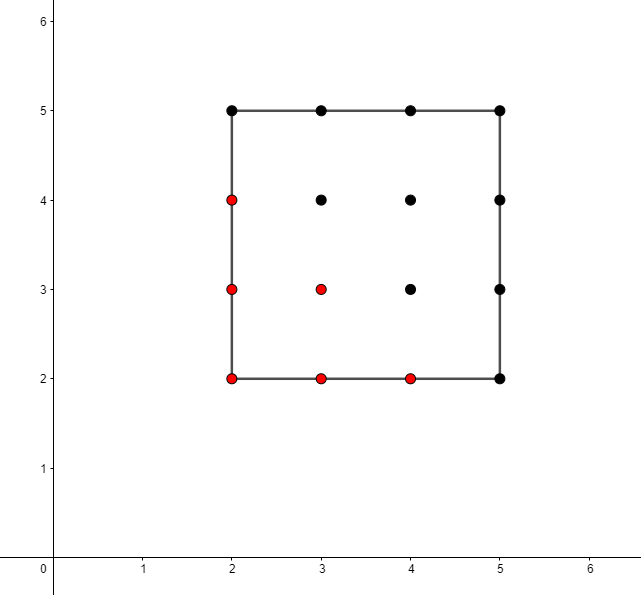Counting Frog Paths
Tag(s):

## Algorithms, Searching

Problem
Editorial
Analytics

There is a frog initially placed at the origin of the coordinate plane. In exactly $1$ second, the frog can either move up $1$ unit, move right $1$ unit, or stay still. In other words, from position $(x,y)$, the frog can spend $1$ second to move to:

• $(x+1,y)$
• $(x,y+1)$
• $(x,y)$

After $T$ seconds, a villager who sees the frog reports that the frog lies on or inside a square of side-length $s$ with coordinates $(X,Y)$, $(X+s,Y)$, $(X,Y+s)$, $(X+s,Y+s)$. Calculate how many points with integer coordinates on or inside this square could be the frog's position after exactly $T$ seconds

Input Format:

The first and only line of input contains four space-separated integers: $X$$Y$$s$, and $T$.

Output Format:

Print the number of points with integer coordinates that could be the frog's position after $T$ seconds.

Constraints:

$0 \le X,Y \le 100$

$1 \le s \le 100$

$0 \le T \le 400$

Note that the Expected Output Feature for Custom Invocation is not supported for this contest.

SAMPLE INPUT
2 2 3 6
SAMPLE OUTPUT
6
ExplanationThe points shown are the points on or in the specified square, and those that are red are the points that the frog could be at after $6$ seconds.

Time Limit: 2.0 sec(s) for each input file.
Memory Limit: 256 MB
Source Limit: 1024 KB
Marking Scheme: Marks are awarded when all the testcases pass.
Allowed Languages: Bash, C, C++, C++14, C++17, Clojure, C#, D, Erlang, F#, Go, Groovy, Haskell, Java, Java 8, Java 14, JavaScript(Rhino), JavaScript(Node.js), Julia, Kotlin, Lisp, Lisp (SBCL), Lua, Objective-C, OCaml, Octave, Pascal, Perl, PHP, Python, Python 3, Python 3.8, R(RScript), Racket, Ruby, Rust, Scala, Swift-4.1, Swift, TypeScript, Visual Basic

## CODE EDITOR

Initializing Code Editor...

## This Problem was Asked inChallenge Name

HourStorm #2

OTHER PROBLEMS OF THIS CHALLENGE
• Math > Counting
• Algorithms > Dynamic Programming